# How to Work Out Area Worksheet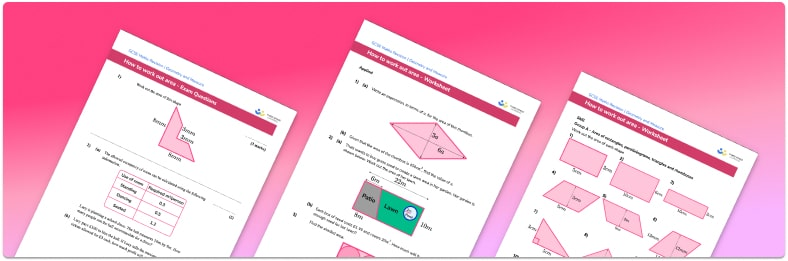Help your students prepare for their Maths GCSE with this free area worksheet of 44 questions and answers

• Section 1 of the printable area worksheet contains 36 skills-based area questions, in 3 groups to support differentiation
• Section 2 contains 4 applied area questions with a mix of worded problems and deeper problem solving questions
• Section 3 contains 4 foundation and higher level GCSE exam style questions
• Answers and a mark scheme for all questions are provided
• Questions follow variation theory with plenty of opportunities for students to work independently at their own level
• All questions created by fully qualified expert secondary maths teachers
• Suitable for GCSE maths revision for AQA, OCR and Edexcel exam boards
• Other worksheets for topics on area, such as areas of a triangle, rectangle, square, parallelogram, and compound shapes, are also available
• To receive this resource and regular emails with more free resources, blog posts and other Third Space updates, enter your email address and click below.

• This field is for validation purposes and should be left unchanged.

You can unsubscribe at any time (each email we send will contain an easy way to unsubscribe). To find out more about how we use your data, see our privacy policy.

### Area at a glance

Area is the amount of space covered by a 2D shape and is measured in square units.

In the simplest cases area can be found by counting the centimetre squares covered by the shape. For the area of irregular shapes involving part-squares, a useful rule for estimating the area is to count all the part-squares which cover half or more of the square centimetres in the shape.

To work out the area of a rectangle, we use the formula length width. The formulae for the area of triangles and the area of parallelograms follow from this. The formula for the area of trapezoids or trapeziums involves adding together the parallel sides, multiplying by the height and dividing by two.

The area of rectilinear shapes (shapes with straight sides and right angles) can be found by splitting the shape into rectangles and finding the area of each one.

It is important to be confident working with decimals and fractions for this topic, in addition to integer lengths and dimensions. Students may also be asked to apply multiplication of fractions to the area of 2D shapes.

When tackling word problems, students are frequently asked to apply these skills to problems such as finding the area of a room or garden space, and then applying additional skills such as calculating a cost to cover or pave that space.

Students can often confuse the perimeter of rectangles and other shapes with finding the area of a rectangle or other shapes. One useful strategy could be to separate these topics in a scheme of work.

Looking forward, students can then progress to finding the area of a circle, or calculating the surface area of prisms. Additional geometry worksheets, for example a 3D shapes printable worksheet or angles in polygons worksheet, are also available.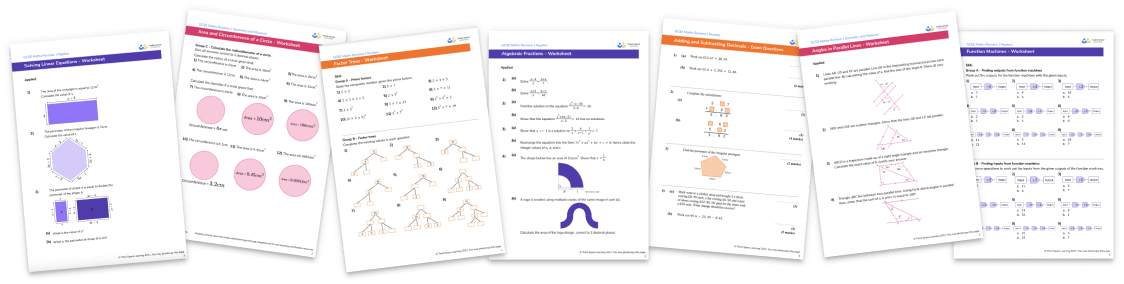For more teaching and learning support on Geometry our GCSE maths lessons provide step by step support for all GCSE maths concepts.

## Related worksheets

Area of a Circle Worksheet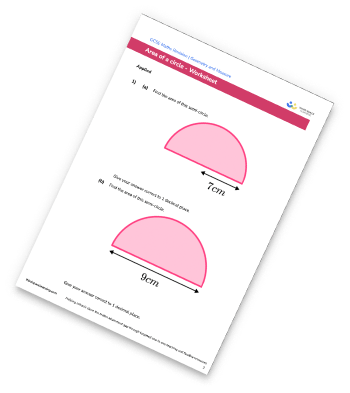Area of Compound Shapes Worksheet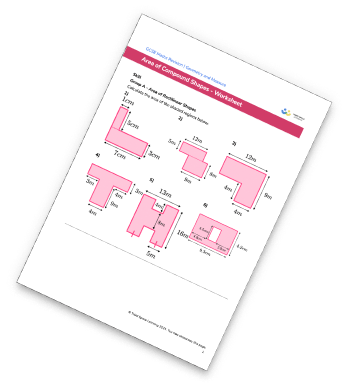Area Of A Trapezium Worksheet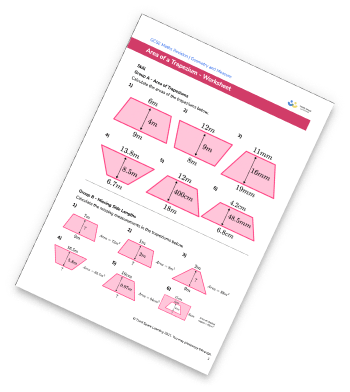Area Of A Rhombus Worksheet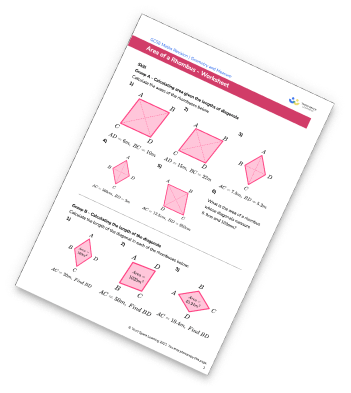## Do you have KS4 students who need more focused attention to succeed at GCSE?There will be students in your class who require individual attention to help them succeed in their maths GCSEs. In a class of 30, it’s not always easy to provide.

Help your students feel confident with exam-style questions and the strategies they’ll need to answer them correctly with our dedicated GCSE maths revision programme.

Lessons are selected to provide support where each student needs it most, and specially-trained GCSE maths tutors adapt the pitch and pace of each lesson. This ensures a personalised revision programme that raises grades and boosts confidence.

Find out more